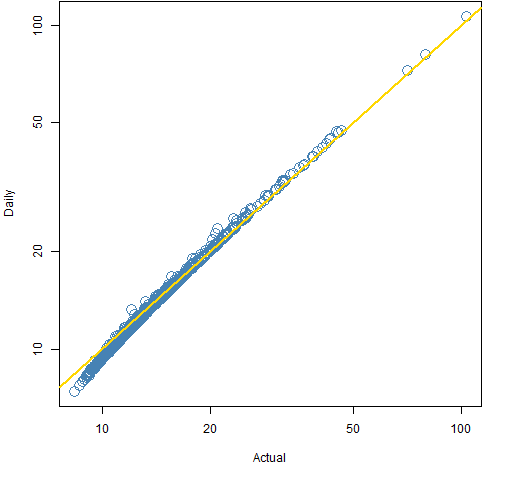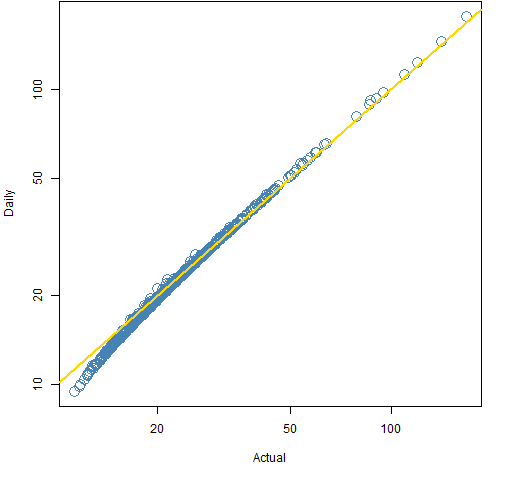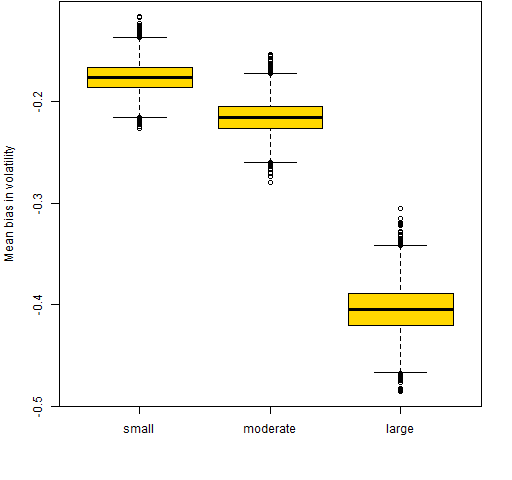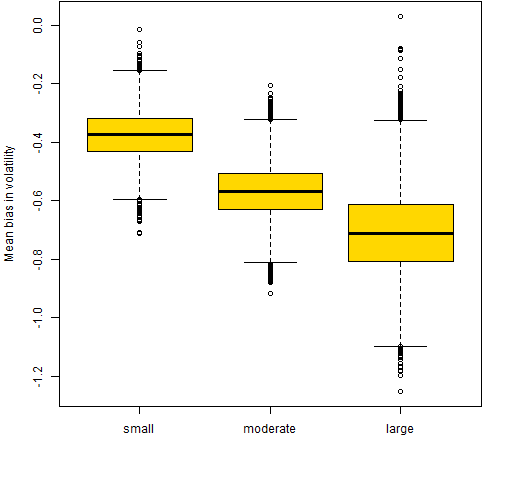# Volatility from daily or monthly: garch evidence

October 29, 2012
By

Want to share your content on R-bloggers? click here if you have a blog, or here if you don't.

Should you use daily or monthly returns to estimate volatility?

Does garch explain why volatility estimated with daily data tends to be bigger than if it is estimated with monthly data?

## Previously

There are a number of previous posts — with the variance compression tag — that discuss the phenomenon of volatility estimated with daily data tending to be higher than if estimated with monthly data.  In particular, there is “The mystery of volatility estimates from daily versus monthly returns”.  Quite apropos of the current post is “The volatility mystery continues”.

There is also “A practical introduction to garch modeling”.

## Experiment

Here’s the idea: Simulate 3 years of daily data from a garch model (of the S&P 500).  Then estimate the volatility over that three years using both the daily returns and the data aggregated into monthly returns.  The key thing with a garch simulation is that we also have the true value of the volatility (unlike market volatility which we never get to see directly).

Three simulations of 1000 series each were performed.  The difference between the simulations was the starting volatility:

• average volatility (and hence moderate)
• small (the 1% quantile of volatility of a long history)
• large (the 99% quantile)

## Results

### Precision

There is a striking difference between the accuracy of volatility estimated with daily data versus monthly data.  Figures 1 and 2 show the actual volatility versus the estimates for the moderate volatility simulation.

Figure 1: Volatility estimates from daily data versus true volatility in the moderate volatility simulation.Figure 2: Volatility estimates from monthly data versus true volatility in the moderate volatility simulation.The plots for the small and large volatility simulations (Figures 3 through 6) tell the same story.

Figure 3: Volatility estimates from daily data versus true volatility in the small volatility simulation.Figure 4: Volatility estimates from monthly data versus true volatility in the small volatility simulation.Figure 5: Volatility estimates from daily data versus true volatility in the large volatility simulation.Figure 6: Volatility estimates from monthly data versus true volatility in the large volatility simulation.### Bias

Figures 7 and 8 show the bootstrap distributions of the mean bias of the daily and monthly estimates in the three simulations.  The bias is of volatility expressed in percent.

Figure 7: Bootstrap distributions of the mean of daily estimate minus actual volatility.Figure 8: Bootstrap distributions of the mean of monthly estimate minus actual volatility.The bias in the monthly estimates is less than one percentage point, and the difference between daily estimates and monthly estimates is even less than that.

## Limitations

The garch model does not exactly replicate how markets operate.  But for these purposes it is probably pretty close.

## Questions

Are there particular reasons to be suspicious of these results?

What is the explanation for the daily estimates to be biased downward for very small actual volatilities?

## Summary

For a garch model the daily estimates of volatility are clearly more accurate than the monthly estimates.  It seems hard to believe that the model is wrong enough to overthrow that in reality.

The garch simulations do exhibit a small amount of variance compression (monthly estimates are smaller than daily).  However, it doesn’t look like there is as much in the garch model as in market data.  I think there is something else happening in the market as well.

## Appendix R

#### garch estimation

See the `rugarch` section of “A practical introduction to garch modeling” for how the garch model was estimated.  It was a garch(1,1) with t-distributed errors.

It is like the one shown in Appendix R of “The volatility mystery continues”.

#### garch simulation

To simulate using the average volatility:

```spxgsim0 <- ugarchsim(spxgar, n.sim=756, m.sim=1000,
startMethod="unconditional")```

`startMethod="sample"`

(which seems like a confusing name for the choice to me).

```spxgsim01 <- ugarchsim(spxgar, n.sim=756, m.sim=1001,
presigma=quantile(spxgarvol, .01)/100 / sqrt(252))```

This command includes an attempt to save me from myself. Slightly different numbers (1000, 1001, 1002) are used in the three simulations.  This can sometimes signal that something is wrong if objects are inappropriately mixed.

#### extract from simulation objects

The (non-transferable) trick of getting relevant data out of `rugarch` simulation objects is to use `as.data.frame`:

```spxgsim0s <- as.data.frame(spxgsim0, which="series")
spxgsim0v <- as.data.frame(spxgsim0, which="sigma")```

#### volatility estimation

The two objects created just above are the inputs to the function that gives us our comparison of volatilities:

```pp.volcompare <- function(series, sigma, month=21)
{
# compare volatility estimates
# placed in public domain 2012 by Burns Statistics
#
# testing status:
# slightly tested, in that monthly returns check
# results seem to make sense

series <- as.matrix(series)
sigma <- as.matrix(sigma)
stopifnot(all(dim(series) == dim(sigma)),
nrow(series) %% month == 0)

ans <- array(NA, c(ncol(series), 3), list(NULL,
c("Daily", "Monthly", "Actual")))
ans[, "Daily"] <- apply(series, 2, sd) * sqrt(252)
ans[, "Actual"] <- sqrt(colSums(sigma^2) *
252/nrow(sigma))
nmon <- nrow(series) / month
mseries <- crossprod(kronecker(diag(nmon),
rep(1, month)), series)
ans[, "Monthly"] <- apply(mseries, 2, sd) *
sqrt(252/month)
ans
}```

The bit with `kronecker` is some matrix magic that gets us the monthly returns that we want.  One hint about how this works is that

`diag(36)`

is the R way of getting the 36 by 36 identity matrix.

Another way of collapsing to monthly returns that is more flexible is:

`crossprod(diag(36)[rep(1:36, each=21),], series)`

We can generalize this formulation to months that have different numbers of days.

#### use the compare function

The function is actually used like:

`spxgsim0comp <- pp.volcompare(spxgsim0s, spxgsim0v)`

The results look like:

```> head(spxgsim0comp)
Daily   Monthly    Actual
[1,] 0.09791878 0.1441274 0.1057857
[2,] 0.21882895 0.2677690 0.2176373
[3,] 0.14704830 0.1454526 0.1499731
[4,] 0.15218896 0.1361271 0.1541258
[5,] 0.14157192 0.1166982 0.1454488
[6,] 0.15529995 0.2052485 0.1573691```

#### bootstrap mean bias

The bootstrapping does a little preparatory work:

```diff0da <- (spxgsim0comp[, "Daily"] -
spxgsim0comp[, "Actual"]) * 100
diff0ma <- (spxgsim0comp[, "Monthly"] -
spxgsim0comp[, "Actual"]) * 100```

The bootstrapping proper is:

```boot0da <- numeric(1e4)
for(i in 1:1e4) {
boot0da[i] <- mean(sample(diff0da, 1000, replace=TRUE))
}
boot0ma <- numeric(1e4)
for(i in 1:1e4) {
boot0ma[i] <- mean(sample(diff0ma, 1000, replace=TRUE))
}```

The boxplots are created like:

```boxplot(list(small=boot01da, moderate=boot0da,
large=boot99da), col="gold",
ylab="Mean bias in volatility")```R-bloggers.com offers daily e-mail updates about R news and tutorials about learning R and many other topics. Click here if you're looking to post or find an R/data-science job.
Want to share your content on R-bloggers? click here if you have a blog, or here if you don't.

Tags: , , , ,